Latest Banking jobs   »

# Quantitative Aptitude Quiz For IBPS SO Prelims 2022- 17th December

Directions (1-5): What will come at the place of (?) question mark in the following series?

Q1. 92, 117, 217, 442, 842, ?
(a) 1367
(b) 1397
(c) 1457
(d) 1467
(e) 1497

Q2. ?, 212, 339, 508, 725, 996
(a) 120
(b) 121
(c) 116
(d) 115
(e) 125

Q3. 4, 6, 9, 13.5, 20.25, ?
(a) 30.375
(b) 30.275
(c) 29.375
(d) 32.375
(e) 32.275

Q4. 81, ? , 155, 276, 445, 734
(a) 105
(b) 104
(c) 106
(d) 110
(e) 112

Q5. 5986, 2996, 1502, 756, ? , 199
(a) 264
(b) 284
(c) 354
(d) 374
(e) 384

Directions (6-10): In each of these questions, two equations (I) and (II) are given. You have to solve both the equations and give answer

Q6. I. x²-9x+20=0
II. y²-11y+30=0
(a) x≤y
(b) x>y
(c) x≥y
(d) x<y
(e) x=y or no relation.

Q7. I. 2x²+13x+6=0
II. y²+19y+90=0
(a) x=y or no relation.
(b) x≥y
(c) x<y
(d) x>y
(e) x≤y

Q8. I. 40x²-13x-42=0
II. 56y²-23y-63=0
(a) x>y
(b) x<y
(c) x≥y
(d) x≤y
(e) x=y or no relation.

Q9. I. (x+11)²=2209
II. (y+19)²=289
(a) x≤y
(b) x=y or no relation.
(c) x<y
(d) x>y
(e) x≥y

Q10. I.4x²-12x-7=0
II. y²-11y+28=0
(a) x> y
(b)y>x
(c)x≥y
(d) y≥x
(e) x=y or no relation.

Q11. Mixture A contains only liquid X and liquid Y while mixture B contains 80% liquid X, 4% liquid Y and 16% liquid Z. If both the mixtures are mixed to get a new mixture having 74% liquid X, 16% liquid Y and 10% liquid Z then find the ratio in which mixture A and mixture B is mixed to get that new mixture?
(a) 5: 6
(b) 3: 2
(c) 2: 3
(d) 3: 5
(e) 5: 7

Q12. Ratio of cost price to that of marked price is 3: 5 and ratio of loss to that of discount is 1:4 then find the discount % given?
(a) 48⅓%
(b) 53⅓%
(c) 58⅓%
(d) 63⅓%
(e) 60 %

Q13. Find the number of 7-digit numbers which can be formed by using all digits 6,5,4,5,3,4,3 only once such that the odd digits occupy odd places only?
(a) 24
(b) 15
(c) 18
(d) 20
(e) 30

Q14. On a certain sum and at the certain rate of interest, CI at the end of two years is Rs 252 while CI at the end of four years is Rs 556.92. Find that amount?
(a) Rs 1000
(b) Rs 1500
(c) Rs 1600
(d) Rs 1800
(e) Rs 1200

Q15. The sum of three consecutive odd numbers and three consecutive even numbers together is 231 and the smallest even number is 9 less than that of smallest odd number. Find the sum of largest odd and largest even number?
(a) 81
(b) 79
(c) 83
(d) 77
(e) 75

Solutions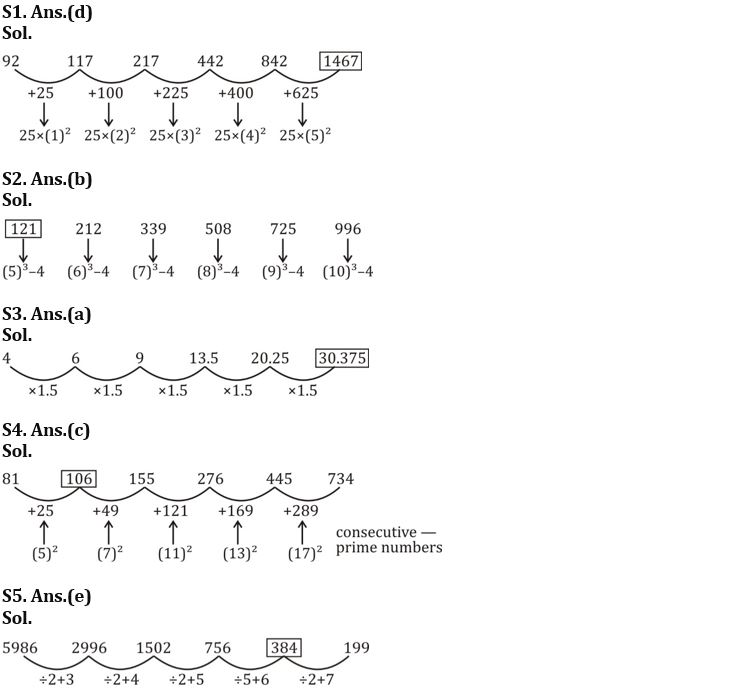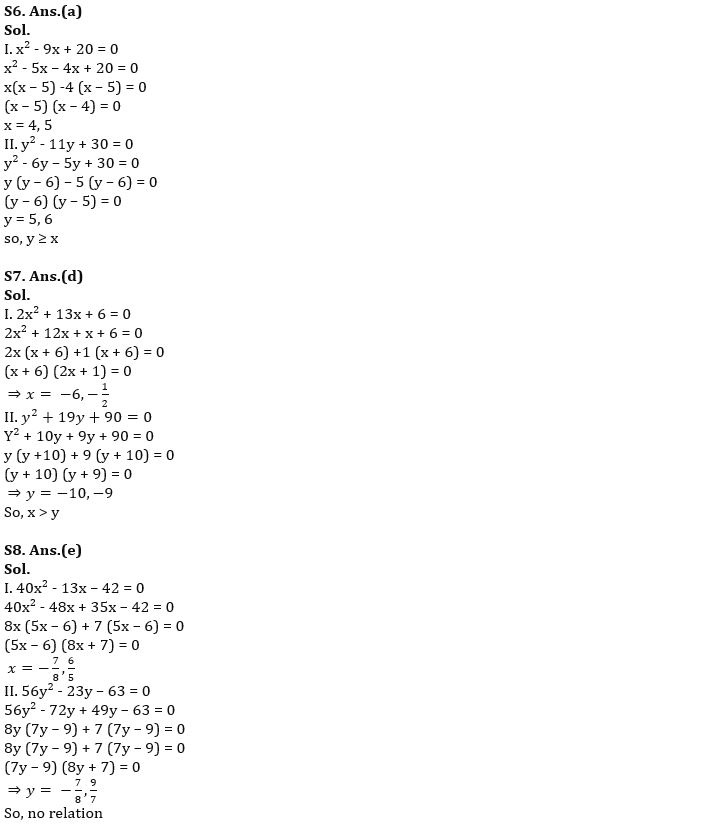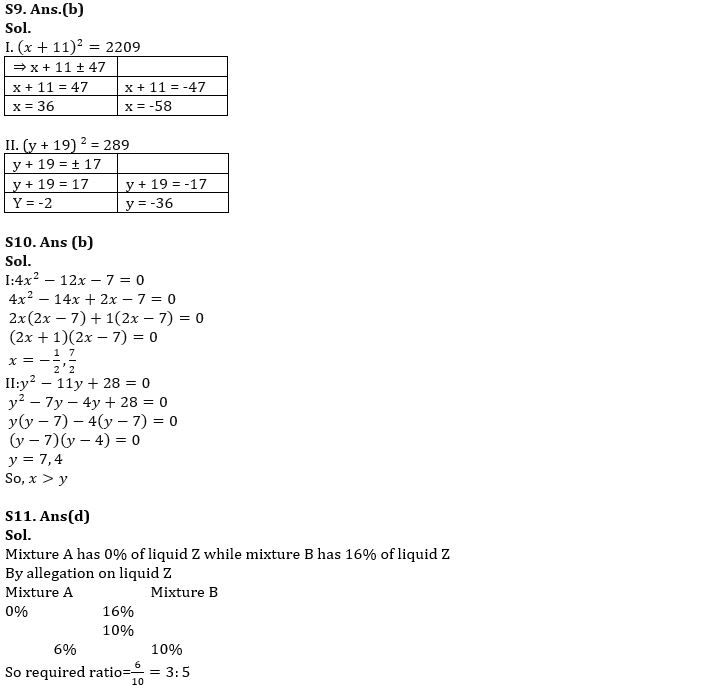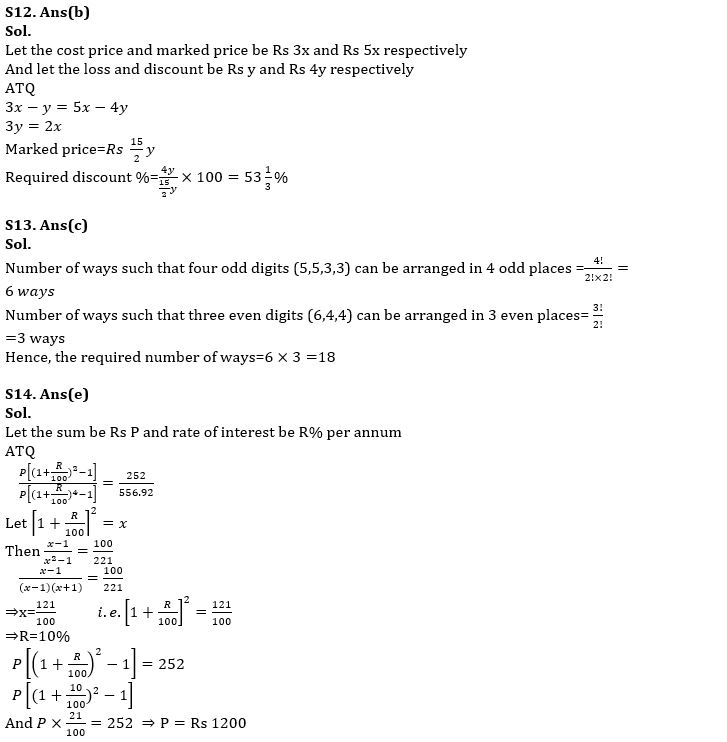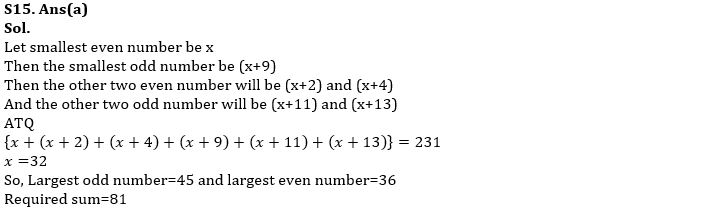#### Congratulations!Union Budget 2023-24: Free PDF